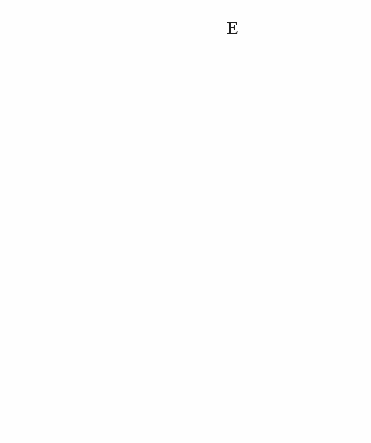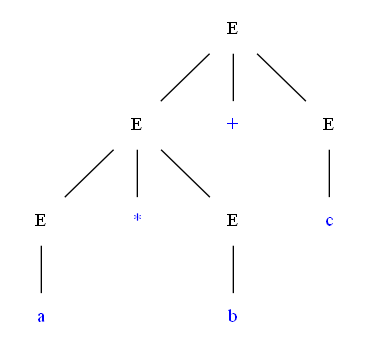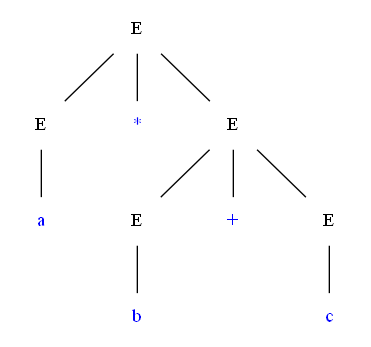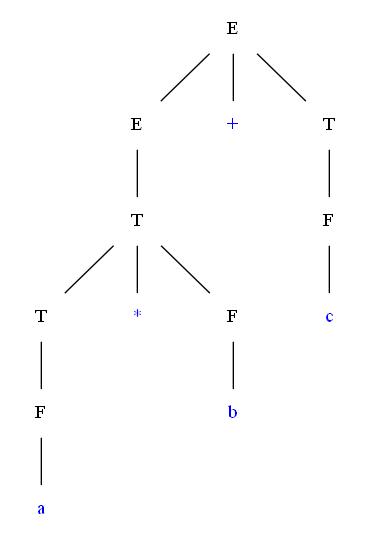IT货架 > > 正文

30

E  =>  E + E  =>  (E) + E  =>  (E + E) + E  =>  (a + E) + E  =>  (a + b) + E  =>  (a + b) + c

E  =>  E + E  =>  E + c  =>  (E) + c  =>  (E + E) + c  =>  (E + b) + c  =>  (a + b) + c\$\$^\$^
 E → idE → E + EE → ( E )
 最左推导 最右推导E → idE → E + EE → E * EE → ( E )

 推导1 推导2F → idF → ( E )T → T * FT → FE → E + T E → T